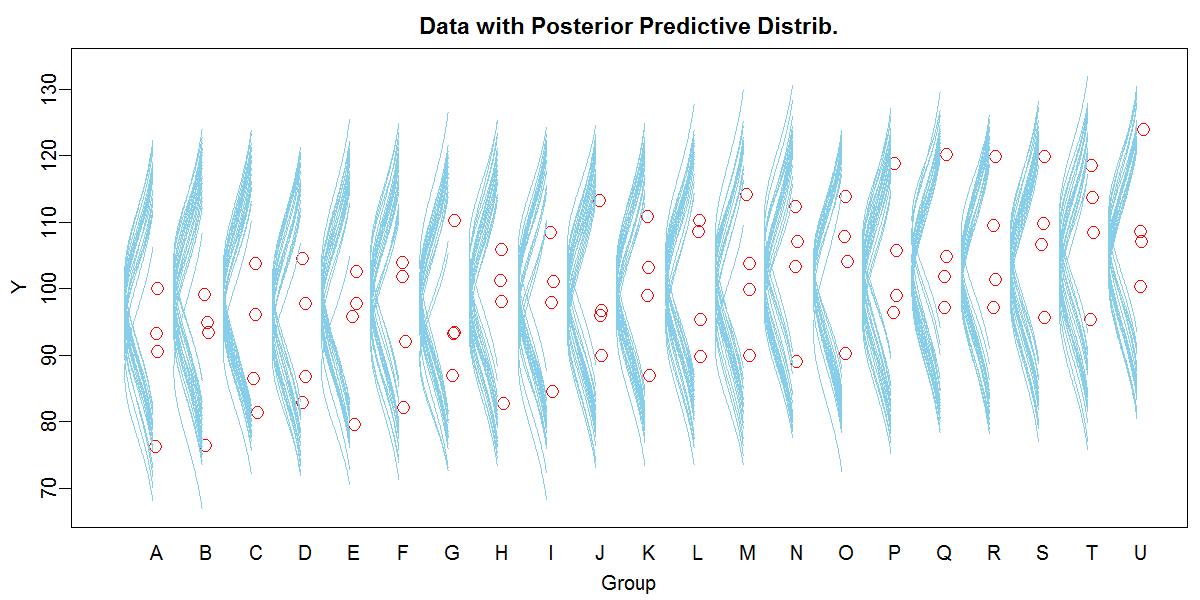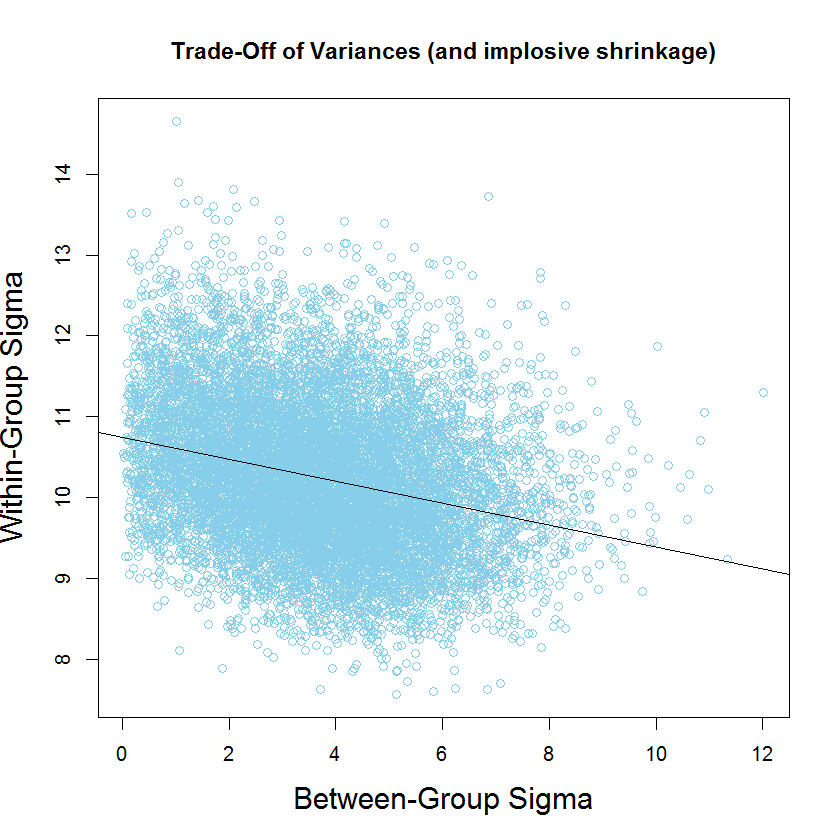## Saturday, April 8, 2017

### Trade-off of between-group and within-group variance (and implosive shrinkage)

Background: Consider data that would traditionally be analyzed as single-factor ANOVA; that is, a continuous metric predicted variable, $y$, and a nominal predictor, "Group." In particular, consider the data plotted as red dots here:A Bayesian approach easily allows a hierarchical model in which both the within-group and between-group variance are estimated. The hierarchical structure imposes shrinkage on the estimated group means. All of this explained in Chapter 19 of DBDA2E

The data above come from Exercise 19.1, which is designed to illustrate "implosive shrinkage." Because there are only a few data points in each group, and there are lots of groups with little variance from baseline, a reasonable description of the data merely collapses the group means close to baseline while expanding the estimate of within-group variance.

The purpose of the present post is to show the trade-off in the posterior distribution of the estimated within-group variance and between-group variance, while also providing another view of implosive shrinkage.

After running the data through the generic hierarchical model in Exercise 19.1, we can look at the posterior distribution of the within-group and between-group standard deviations. The code below produces the following plot:

mcmcMat = as.matrix( mcmcCoda )
openGraph()
plot( mcmcMat[,"aSigma"] , mcmcMat[,"ySigma"] ,
xlab="Between-Group Sigma" , ylab="Within-Group Sigma" ,
col="skyblue" , cex.lab=1.5 ,
main="Trade-Off of Variances (and implosive shrinkage)" )
abline( lm( mcmcMat[,"ySigma"] ~ mcmcMat[,"aSigma"] ) )Notice in the plot that as the between-group standard deviation gets larger, the within-group standard deviation gets smaller. Notice also the implosive shrinkage: The estimate of between-group standard deviation is "piled up" against zero.

1.Hello, just starting this chapter and encountered this error below. Is it something obvious I have misspelt? Thanks, Graeme

yName="Longevity"
xName="CompanionNumber"
source("Jags-Ymet-Xnom1fac-MnormalHom.R")
mcmcCoda=genMCMC(datFrm=myDataFrame,yName=yName, xName=xName,
numSavedSteps=1100, thinSteps=10, saveName=fileNameRoot)

Error in genMCMC(datFrm = myDataFrame, yName = yName, xName = xName, numSavedSteps = 1100, :

OUTPUT
. Initializing model
-------------------------------------------------| 500
++++++++++++++++++++++++++++++++++++++++++++++++++ 100%
. Updating 1000
-------------------------------------------------| 1000
************************************************** 100%
. . . . . . Updating 3670
-------------------------------------------------| 3650
************************************************** 100%
* 100%
. . . . Updating 0
. Deleting model
.
All chains have finished
Finished running the simulation

1.In the high-level script, one of the lines defines fileNameRoot. Be sure you've executed that line.

2.Hi John:
What is the difference between your model and using a so-called random effect ANOVA using lme4 or nlme, both of which are frequentist? The shrinkage here is also obtained by bayes theorem for the random effects. If you summarize the posterior with the mode, I would imagine the estimates are equivalent to those from a frequentist model, which also uses the mode.

1.Don: Shrinkage comes from the hierarchical model, not from Bayesian estimation. A maximum likelihood estimate of the hierarchical model would also show shrinkage. A major benefit of the Bayesian approach is the explicit posterior distribution on all the parameters -- no need for auxiliary assumptions to generate sampling distributions for p values and confidence intervals. (I'd have to delve into the details of lme4 to know if it's the very same model structure -- might be. But in JAGS, it's easy to generalize to non-normal distributions and heterogeneous variances.)